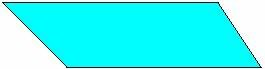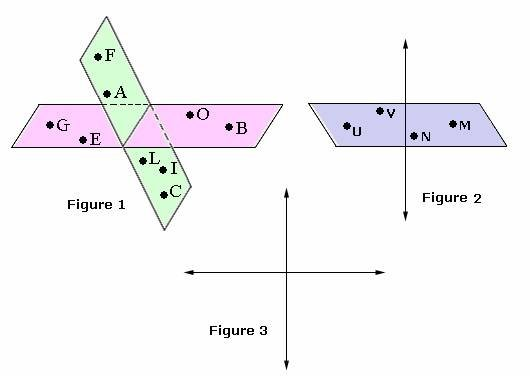Definition Of Plane

A flat surface that extends into infinity in all directions is known as a Plane.

It is also called as two-dimensional surface.
Any three noncollinear points lie on one and only one plane.
A plane has infinite width and length, zero thickness, and zero curvature.

Examples of PlaneThe figure shown is a flat surface extended in all the directions. So, it is a plane.

Solved Example on Plane

Ques: Which of the figures represents that one plane is being cut by another?Choices:

A. Figure 1
B. Figure 3
C. Figure 2
D. None of the above